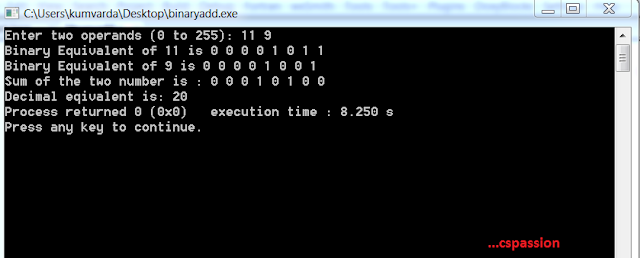## 8-Bit Binary Adder program in C

On 23:37 by Vardan Kumar in ,    No comments

## 8-Bit Binary Addition in C

Binary number system is the system which is used by the computer, it is the key for really fast mathematical computations performed, so let us discuss the logic for 8-bit binary adder step by step.

### Prerequisites

Above two logic understandings are really important in order to make the inputs and outputs user as well as computer understandable. We'll discuss one full logic in here but it is recommended that you go for step by step explanation by checking out aove mentioned links.

### Logic

• Let the user input two operands, as we are dealing with 8-bit format so restrict the input from 0-255.
• Convert the two operands into its binary equivalent and store the results in two separate arrays. Displaying binary equivalent to user is optional but advised.
• Pass the two arrays and the result array as arguments to the function that would do the core computation of adding 8-bit binary numbers.
• Loop bit by bit till 8 bits i.e. from 0 to 7.
• Declaring initial carry bit as 0, do a^b^c and store the result bit in result array, where a is first input array, b is second input array and c is carry
• Evaluate the carry bit by performing ab+bc+ca i.e. (a&b) | (b&c) | (c&a).
• The result array is updated with the final result as the loop terminates.
• Return the carry bit 'c'.
• Check if the carry bit is 1 or 0.
• If it is 1 then print overflow since we are dealing with 8-bit addition and if carry at the end of computation is still 1 then the result would be 9 bits.
• If it is 0 then print the result as binary number and it is recommended to convert the 8 -bit result to its decimal equivalent.

### Understanding With Example

• Suppose the input numbers are 11 and 9.
• 8-bit Binary Equivalents: 11-> 00001011 and 9-> 00001001
• c=0,a=1 and b=1, performing result=a^b^c(1^1^0)=0,(X-or returns 0 if two operands are same and 1 if two operands are different,so 1^1=0,1^0=1).
• Carry bit=ab+bc+ca i.e (1&1)+(1&0)+(1&0)=1,(And(&) returns 0 if any of the two bits is 0 and Or(|) returns 1 if any of two bits is 1)
• c=1,a=1,b=0, performing result=a^b^c(1^0^1)=0
• Carry bit=ab+bc+ca i.e (1&0)|(0&1)|(1&1)=1
• c=1,a=0,b=0, performing result=a^b^c(0^0^1)=1
• Carry Bit=ab+bc+ca i.e (0&0)|(0&1)|(1&0)=0
• c=0,a=1,b=1, performing result=a^b^c(1^1^0)=0
• Carry Bit=ab+bc+ca i.e (1&1)|(1&0)|(0&1)=1
• c=1,a=0,b=0,performing a^b^c(0^0^1)=1
• carry Bit=ab+bc+ca i.e (0&0)|(0&1)|(1&0)=0
• Rest all the result bits will be 0.
• Final result array will be 00010100.
• Converting to its decimal equivalent will give 20(11+9).8-Bit binary adder output

### Source Code

#include <stdio.h>
#include <math.h>

void decimal2Binary(int op1, int aOp[]){
int result, i = 0;
do{
result = op1 % 2;
op1 /= 2;
aOp[i] = result;
i++;
}while(op1 > 0);
}

int binary2Decimal(int array[]){
int sum = 0, i;
for(i = 0; i < 8; i++){
if(array[i]) sum += pow(2,i);
}
return sum;
}

void displayBinary(int array[], int n){
int i;
for(i = n -1; i >=0; i--){
printf("%d ", array[i]);

}
printf("\n");
}

int addBinary(int a1[], int a2[], int result[]){
int i, c = 0;
for(i = 0; i < 8 ; i++){
result[i] = ((a1[i] ^ a2[i]) ^ c); //a xor b xor c
c = ((a1[i] & a2[i]) | (a1[i] &c)) | (a2[i] & c); //ab+bc+ca
}
result[i] = c;
return c;
}

int main(){
int op1, op2, sum;
int  a = {0,0,0,0,0,0,0,0};
int  b = {0,0,0,0,0,0,0,0};
int  aSum = {0,0,0,0,0,0,0,0};
printf("Enter two operands (0 to 255): ");
scanf("%d %d", &op1, &op2);
while(op1 < 0 || op1 > 255 || op2 < 0 || op2 > 255 ){
printf("Enter two operands (0 to 255): ");
scanf("%d %d", &op1, &op2);
}
decimal2Binary(op1, a);
decimal2Binary(op2, b);
printf("Binary Equivalent of %d is ",op1);
displayBinary(a, 8);
printf("Binary Equivalent of %d is ",op2);
displayBinary(b, 8);